# RD Sharma Solutions for Class 10 Maths Chapter 9 Arithmetic Progressions Exercise 9.1

Sequences are observed all around us in everyday life. In this chapter, we are specifically concentrating on one type of sequences known as the arithmetic progressions. The first exercise of this chapter 9 is an introductory exercise to writing down terms of a sequence. For a clear understanding of this basics you can access the RD Sharma Solutions for Class 10 Maths Chapter 9 Arithmetic Progressions Exercise 9.1 PDF provided below for detailed solutions to problems.

## RD Sharma Solutions for Class 10 Maths Chapter 9 Arithmetic Progressions Exercise 9.1 Download PDF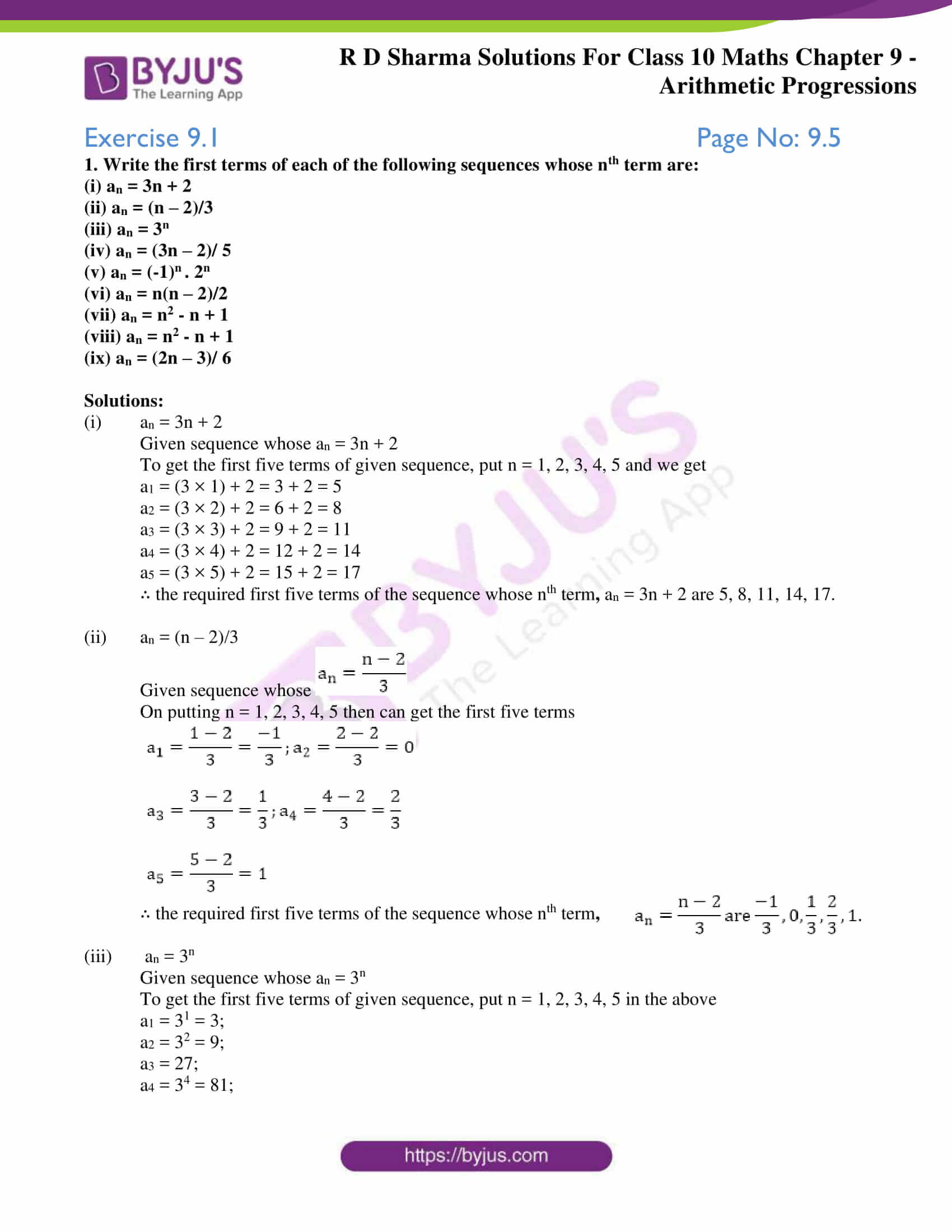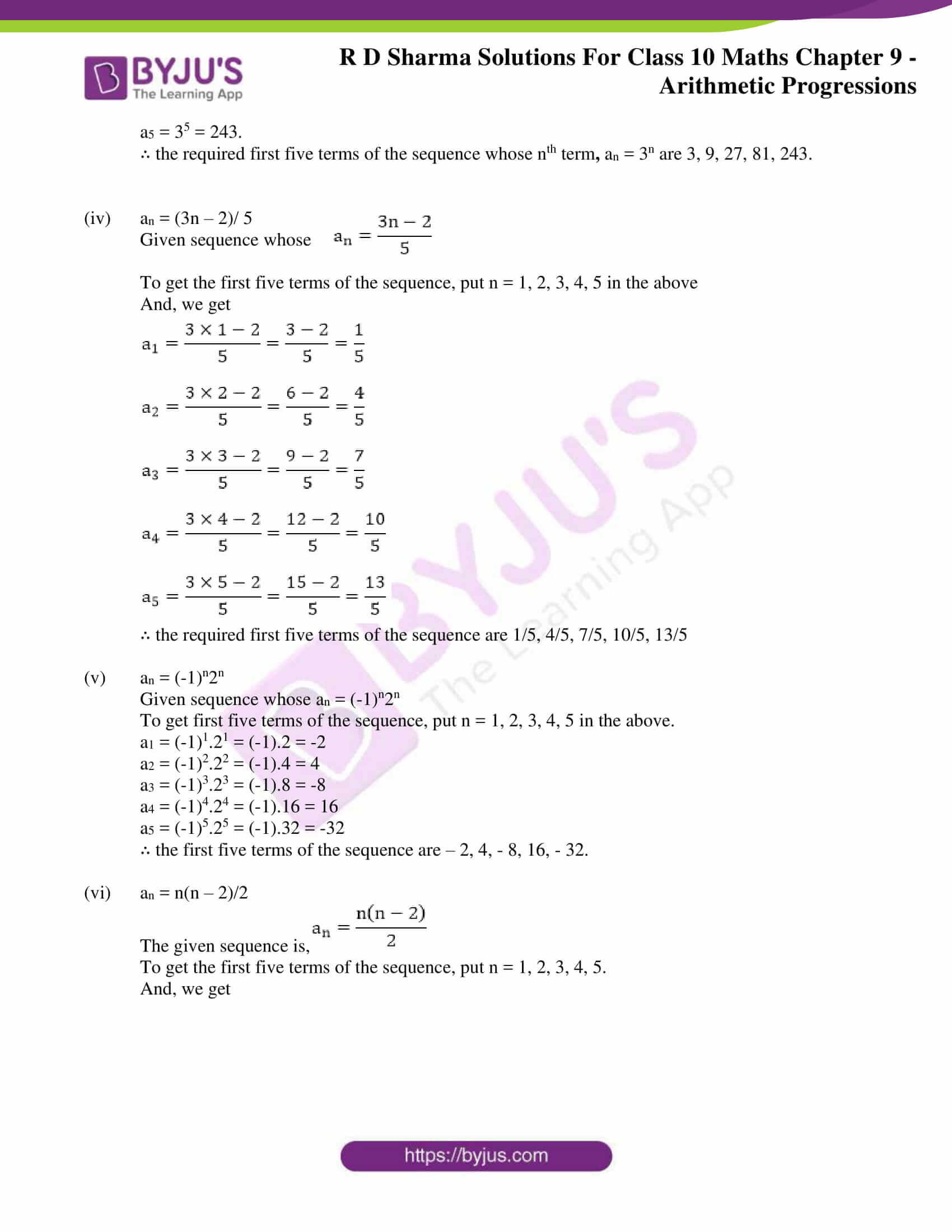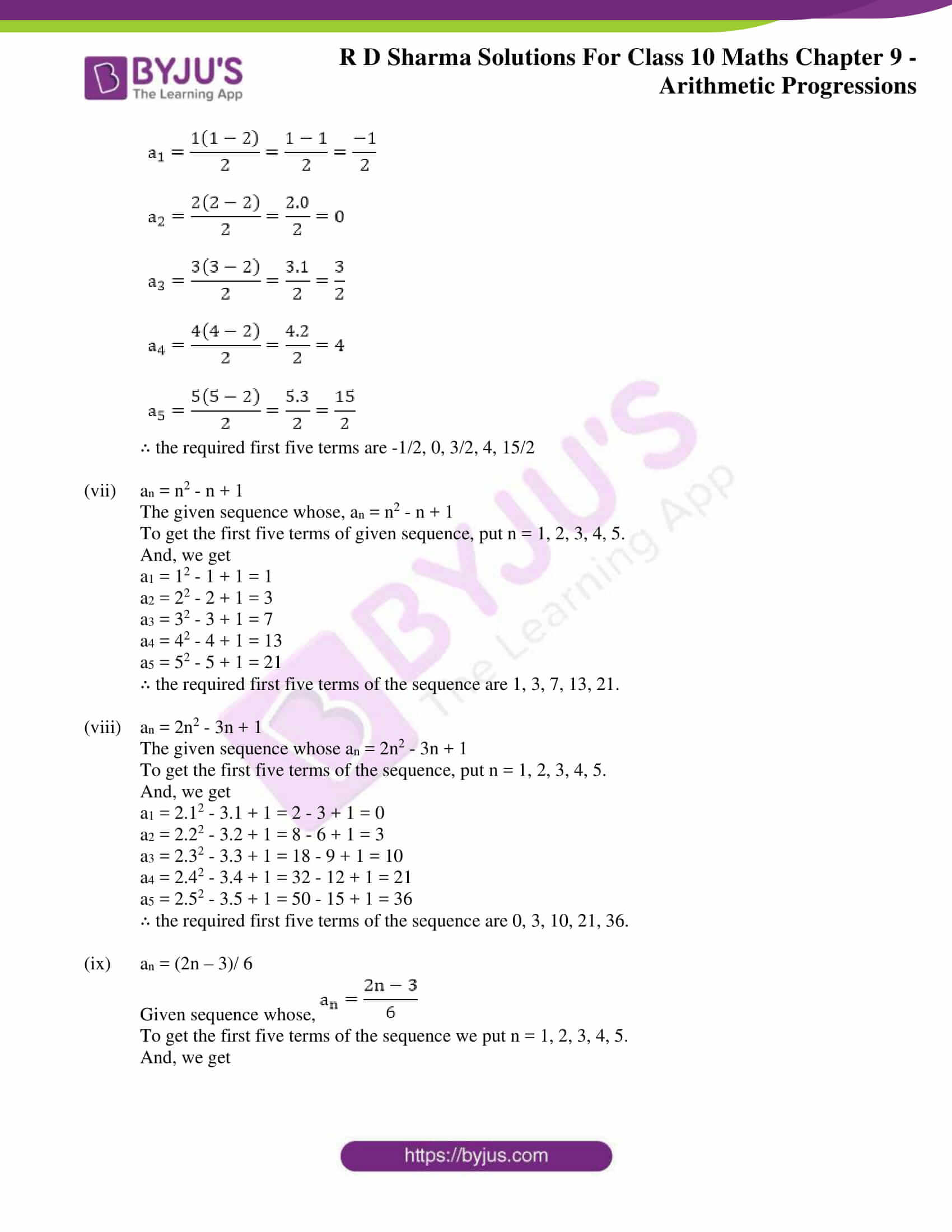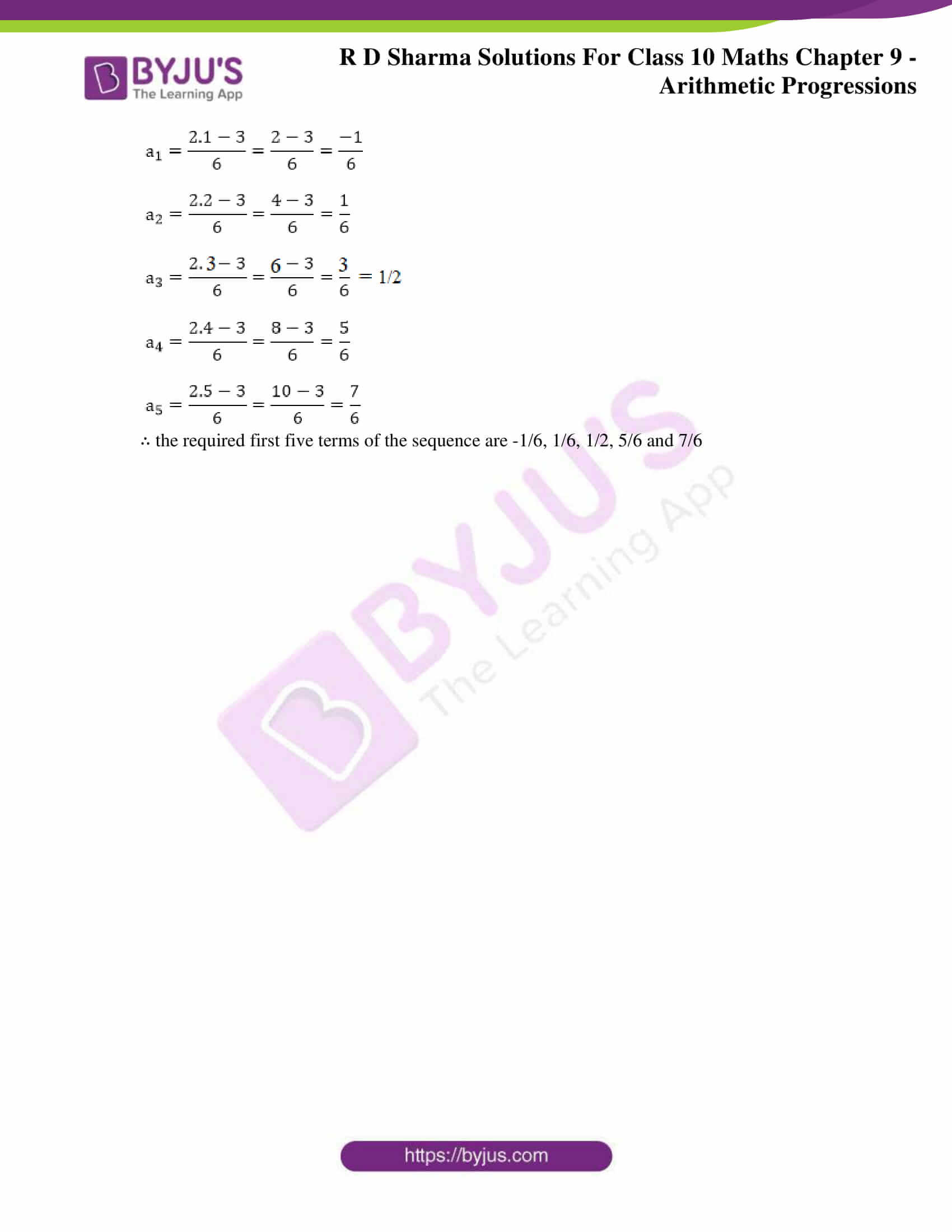### Access RD Sharma Solutions for Class 10 Maths Chapter 9 Arithmetic Progressions Exercise 9.1

1. Write the first terms of each of the following sequences whose nth term are:

(i) an = 3n + 2

(ii) an = (n – 2)/3

(iii) an = 3n

(iv) an = (3n – 2)/ 5

(v) an = (-1)n . 2n

(vi) an = n(n – 2)/2

(vii) an = n2 – n + 1

(viii) an = n2 – n + 1

(ix) an = (2n – 3)/ 6

Solutions:

(i) an = 3n + 2

Given sequence whose an = 3n + 2

To get the first five terms of given sequence, put n = 1, 2, 3, 4, 5 and we get

a1 = (3 × 1) + 2 = 3 + 2 = 5

a2 = (3 × 2) + 2 = 6 + 2 = 8

a3 = (3 × 3) + 2 = 9 + 2 = 11

a4 = (3 × 4) + 2 = 12 + 2 = 14

a5 = (3 × 5) + 2 = 15 + 2 = 17

∴ the required first five terms of the sequence whose nth term, an = 3n + 2 are 5, 8, 11, 14, 17.

(ii) an = (n – 2)/3

Given sequence whose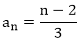On putting n = 1, 2, 3, 4, 5 then can get the first five terms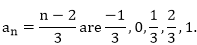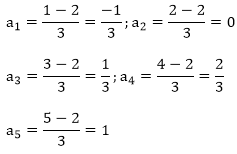∴ the required first five terms of the sequence whose nth term,

(iii) an = 3n

Given sequence whose an = 3n

To get the first five terms of given sequence, put n = 1, 2, 3, 4, 5 in the above

a1 = 31 = 3;

a2 = 32 = 9;

a3 = 27;

a4 = 34 = 81;

a5 = 35 = 243.

∴ the required first five terms of the sequence whose nth term, an = 3n are 3, 9, 27, 81, 243.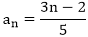(iv) an = (3n – 2)/ 5

Given sequence whose

To get the first five terms of the sequence, put n = 1, 2, 3, 4, 5 in the above

And, we get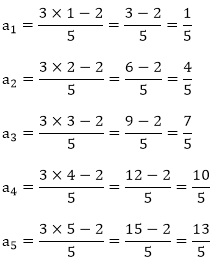∴ the required first five terms of the sequence are 1/5, 4/5, 7/5, 10/5, 13/5

(v) an = (-1)n2n

Given sequence whose an = (-1)n2n

To get first five terms of the sequence, put n = 1, 2, 3, 4, 5 in the above.

a1 = (-1)1.21 = (-1).2 = -2

a2 = (-1)2.22 = (-1).4 = 4

a3 = (-1)3.23 = (-1).8 = -8

a4 = (-1)4.24 = (-1).16 = 16

a5 = (-1)5.25 = (-1).32 = -32

∴ the first five terms of the sequence are – 2, 4, – 8, 16, – 32.

(vi) an = n(n – 2)/2

The given sequence is,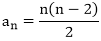To get the first five terms of the sequence, put n = 1, 2, 3, 4, 5.

And, we get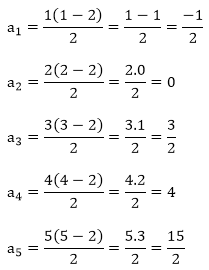∴ the required first five terms are -1/2, 0, 3/2, 4, 15/2

(vii) an = n2 – n + 1

The given sequence whose, an = n2 – n + 1

To get the first five terms of given sequence, put n = 1, 2, 3, 4, 5.

And, we get

a1 = 12 – 1 + 1 = 1

a2 = 22 – 2 + 1 = 3

a3 = 32 – 3 + 1 = 7

a4 = 42 – 4 + 1 = 13

a5 = 52 – 5 + 1 = 21

∴ the required first five terms of the sequence are 1, 3, 7, 13, 21.

(viii) an = 2n2 – 3n + 1

The given sequence whose an = 2n2 – 3n + 1

To get the first five terms of the sequence, put n = 1, 2, 3, 4, 5.

And, we get

a1 = 2.12 – 3.1 + 1 = 2 – 3 + 1 = 0

a2 = 2.22 – 3.2 + 1 = 8 – 6 + 1 = 3

a3 = 2.32 – 3.3 + 1 = 18 – 9 + 1 = 10

a4 = 2.42 – 3.4 + 1 = 32 – 12 + 1 = 21

a5 = 2.52 – 3.5 + 1 = 50 – 15 + 1 = 36

∴ the required first five terms of the sequence are 0, 3, 10, 21, 36.

(ix) an = (2n – 3)/ 6

Given sequence whose,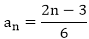To get the first five terms of the sequence we put n = 1, 2, 3, 4, 5.

And, we get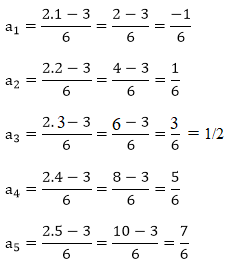∴ the required first five terms of the sequence are -1/6, 1/6, 1/2, 5/6 and 7/6